# Part 1: Overview of last Classes

Download new data set, frequency of several diseases in Boston since the 1880’s BosEpi.tab.txt

``bosEpi = read.delim(file.choose())``

View data table

``View(bosEpi)``

Use the `str()` and `summary()` to get a quick summary of the data

``str(bosEpi)``
``````'data.frame':   30062 obs. of  8 variables:
\$ disease: Factor w/ 23 levels "BRUCELLOSIS [UNDULANT FEVER]",..: 20 5 22 20 5 22 20 5 20 16 ...
\$ event  : Factor w/ 2 levels "CASES","DEATHS": 2 2 2 2 2 2 2 2 2 2 ...
\$ number : int  4 7 2 2 5 2 5 8 2 1 ...
\$ loc    : Factor w/ 1 level "BOSTON": 1 1 1 1 1 1 1 1 1 1 ...
\$ state  : Factor w/ 2 levels "IN","MA": 2 2 2 2 2 2 2 2 2 2 ...
\$ Year   : int  1888 1888 1888 1888 1888 1888 1888 1888 1888 1888 ...
\$ Month  : int  7 7 7 7 7 7 8 8 8 8 ...
\$ Day    : int  22 22 22 29 29 29 5 5 12 12 ...``````
``summary(bosEpi)``
``````                               disease         event
DIPHTHERIA                        : 3866   CASES :14620
TUBERCULOSIS [PHTHISIS PULMONALIS]: 3479   DEATHS:15442
TYPHOID FEVER [ENTERIC FEVER]     : 3312
SCARLET FEVER                     : 3282
MEASLES                           : 3164
PNEUMONIA AND INFLUENZA           : 2276
(Other)                           :10683
number            loc        state           Year
Min.   :   0.00   BOSTON:30062   IN:    1   Min.   :1888
1st Qu.:   1.00                  MA:30061   1st Qu.:1913
Median :   7.00                             Median :1925
Mean   :  21.68                             Mean   :1927
3rd Qu.:  25.00                             3rd Qu.:1936
Max.   :2859.00                             Max.   :2013

Month             Day
Min.   : 1.000   Min.   : 1.00
1st Qu.: 3.000   1st Qu.: 8.00
Median : 6.000   Median :16.00
Mean   : 6.462   Mean   :15.75
3rd Qu.: 9.000   3rd Qu.:23.00
Max.   :12.000   Max.   :31.00
``````

Important columns of the data set
number - Number of occurences
loc - Location of disease
event - Whether the number in number is number of cases or number of deaths from disease
disease - Disease Name
Year - Year of occurence
Month - Month of occurrence
Day - Day of occurrence

## Accesing Data

Can get a column by using `\$` and the name of the column or using `[,]` notation
Also since this is a very large table you can use `head()` to print a manageable amount of information

``head(bosEpi\$Year)``
`` 1888 1888 1888 1888 1888 1888``

or

``head(bosEpi[,6])``
`` 1888 1888 1888 1888 1888 1888``

Get multiple columns using `[]` and `:`
The following will get the columns 6 through 8

``head(bosEpi[,6:8])``
``````  Year Month Day
1 1888     7  22
2 1888     7  22
3 1888     7  22
4 1888     7  29
5 1888     7  29
6 1888     7  29``````

Or get columns by name and `[]`

``head(bosEpi[,c("disease", "number", "event", "Year")])``
``````                        disease number  event Year
1 TYPHOID FEVER [ENTERIC FEVER]      4 DEATHS 1888
2                    DIPHTHERIA      7 DEATHS 1888
3    WHOOPING COUGH [PERTUSSIS]      2 DEATHS 1888
4 TYPHOID FEVER [ENTERIC FEVER]      2 DEATHS 1888
5                    DIPHTHERIA      5 DEATHS 1888
6    WHOOPING COUGH [PERTUSSIS]      2 DEATHS 1888``````

Getting only select rows
For example to get first five rows of bosEpi

``bosEpi[1:5,]``
``````                        disease  event number    loc state
1 TYPHOID FEVER [ENTERIC FEVER] DEATHS      4 BOSTON    MA
2                    DIPHTHERIA DEATHS      7 BOSTON    MA
3    WHOOPING COUGH [PERTUSSIS] DEATHS      2 BOSTON    MA
4 TYPHOID FEVER [ENTERIC FEVER] DEATHS      2 BOSTON    MA
5                    DIPHTHERIA DEATHS      5 BOSTON    MA
Year Month Day
1 1888     7  22
2 1888     7  22
3 1888     7  22
4 1888     7  29
5 1888     7  29``````

Combining getting only select rows and columns

Using column numbers

``bosEpi[1:5,1:3]``
``````                        disease  event number
1 TYPHOID FEVER [ENTERIC FEVER] DEATHS      4
2                    DIPHTHERIA DEATHS      7
3    WHOOPING COUGH [PERTUSSIS] DEATHS      2
4 TYPHOID FEVER [ENTERIC FEVER] DEATHS      2
5                    DIPHTHERIA DEATHS      5``````

Using column names

``bosEpi[1:5,c("disease", "event", "number")]``
``````                        disease  event number
1 TYPHOID FEVER [ENTERIC FEVER] DEATHS      4
2                    DIPHTHERIA DEATHS      7
3    WHOOPING COUGH [PERTUSSIS] DEATHS      2
4 TYPHOID FEVER [ENTERIC FEVER] DEATHS      2
5                    DIPHTHERIA DEATHS      5``````

## Using Logic

Here is a table of logic operators to use on data

operator meaning
< less than
<= less than or equal to
> greater than
>= greater than or equal to
== exactly equal to
!= not equal to

Testing the year column for the year 1888 and looking at the first 50 rows

``head(bosEpi\$Year == 1888, n = 50) ``
``````   TRUE  TRUE  TRUE  TRUE  TRUE  TRUE  TRUE  TRUE  TRUE
  TRUE  TRUE  TRUE  TRUE  TRUE  TRUE  TRUE  TRUE  TRUE
  TRUE  TRUE  TRUE  TRUE  TRUE  TRUE  TRUE  TRUE  TRUE
  TRUE  TRUE  TRUE  TRUE  TRUE  TRUE  TRUE  TRUE  TRUE
  TRUE  TRUE  TRUE  TRUE  TRUE  TRUE  TRUE  TRUE  TRUE
 FALSE FALSE FALSE FALSE FALSE``````

Testing for anything happening before 1890 not including 1890

``head(bosEpi\$Year < 1890, n = 100) ``
``````    TRUE  TRUE  TRUE  TRUE  TRUE  TRUE  TRUE  TRUE  TRUE
  TRUE  TRUE  TRUE  TRUE  TRUE  TRUE  TRUE  TRUE  TRUE
  TRUE  TRUE  TRUE  TRUE  TRUE  TRUE  TRUE  TRUE  TRUE
  TRUE  TRUE  TRUE  TRUE  TRUE  TRUE  TRUE  TRUE  TRUE
  TRUE  TRUE  TRUE  TRUE  TRUE  TRUE  TRUE  TRUE  TRUE
  TRUE  TRUE  TRUE  TRUE  TRUE  TRUE  TRUE  TRUE  TRUE
  TRUE  TRUE  TRUE  TRUE  TRUE  TRUE  TRUE  TRUE  TRUE
  TRUE  TRUE  TRUE  TRUE  TRUE  TRUE  TRUE  TRUE  TRUE
  TRUE  TRUE  TRUE FALSE FALSE FALSE FALSE FALSE FALSE
 FALSE FALSE FALSE FALSE FALSE FALSE FALSE FALSE FALSE
 FALSE FALSE FALSE FALSE FALSE FALSE FALSE FALSE FALSE
 FALSE``````

Testing for anything happening before 1890 including 1890

``head(bosEpi\$Year <= 1890, n = 300) ``
``````    TRUE  TRUE  TRUE  TRUE  TRUE  TRUE  TRUE  TRUE  TRUE
  TRUE  TRUE  TRUE  TRUE  TRUE  TRUE  TRUE  TRUE  TRUE
  TRUE  TRUE  TRUE  TRUE  TRUE  TRUE  TRUE  TRUE  TRUE
  TRUE  TRUE  TRUE  TRUE  TRUE  TRUE  TRUE  TRUE  TRUE
  TRUE  TRUE  TRUE  TRUE  TRUE  TRUE  TRUE  TRUE  TRUE
  TRUE  TRUE  TRUE  TRUE  TRUE  TRUE  TRUE  TRUE  TRUE
  TRUE  TRUE  TRUE  TRUE  TRUE  TRUE  TRUE  TRUE  TRUE
  TRUE  TRUE  TRUE  TRUE  TRUE  TRUE  TRUE  TRUE  TRUE
  TRUE  TRUE  TRUE  TRUE  TRUE  TRUE  TRUE  TRUE  TRUE
  TRUE  TRUE  TRUE  TRUE  TRUE  TRUE  TRUE  TRUE  TRUE
  TRUE  TRUE  TRUE  TRUE  TRUE  TRUE  TRUE  TRUE  TRUE
  TRUE  TRUE  TRUE  TRUE  TRUE  TRUE  TRUE  TRUE  TRUE
  TRUE  TRUE  TRUE  TRUE  TRUE  TRUE  TRUE  TRUE  TRUE
  TRUE  TRUE  TRUE  TRUE  TRUE  TRUE  TRUE  TRUE  TRUE
  TRUE  TRUE  TRUE  TRUE  TRUE  TRUE  TRUE  TRUE  TRUE
  TRUE  TRUE  TRUE  TRUE  TRUE  TRUE  TRUE  TRUE  TRUE
  TRUE  TRUE  TRUE  TRUE  TRUE  TRUE  TRUE  TRUE  TRUE
  TRUE  TRUE  TRUE  TRUE  TRUE  TRUE  TRUE  TRUE  TRUE
  TRUE  TRUE  TRUE  TRUE  TRUE  TRUE  TRUE  TRUE  TRUE
  TRUE  TRUE  TRUE  TRUE  TRUE  TRUE  TRUE  TRUE  TRUE
  TRUE  TRUE  TRUE  TRUE  TRUE  TRUE  TRUE  TRUE  TRUE
  TRUE  TRUE  TRUE  TRUE  TRUE  TRUE  TRUE  TRUE  TRUE
  TRUE  TRUE  TRUE  TRUE  TRUE  TRUE  TRUE  TRUE  TRUE
  TRUE  TRUE  TRUE  TRUE  TRUE  TRUE  TRUE  TRUE  TRUE
  TRUE  TRUE  TRUE  TRUE  TRUE  TRUE  TRUE  TRUE  TRUE
  TRUE  TRUE  TRUE  TRUE  TRUE  TRUE  TRUE  TRUE  TRUE
  TRUE  TRUE  TRUE FALSE FALSE FALSE FALSE FALSE FALSE
 FALSE FALSE FALSE FALSE FALSE FALSE FALSE FALSE FALSE
 FALSE FALSE FALSE FALSE FALSE FALSE FALSE FALSE FALSE
 FALSE FALSE FALSE FALSE FALSE FALSE FALSE FALSE FALSE
 FALSE FALSE FALSE FALSE FALSE FALSE FALSE FALSE FALSE
 FALSE FALSE FALSE FALSE FALSE FALSE FALSE FALSE FALSE
 FALSE FALSE FALSE FALSE FALSE FALSE FALSE FALSE FALSE
 FALSE FALSE FALSE``````

Combining logic with accessing elements of a `data.frame`
Getting the rows that contain data from only the year 1888 by telling R to get rows only where `bosEpi\$Year` is equal to 1888

``bosEpi[bosEpi\$Year == 1888,]``
``````                         disease  event number    loc state
1  TYPHOID FEVER [ENTERIC FEVER] DEATHS      4 BOSTON    MA
2                     DIPHTHERIA DEATHS      7 BOSTON    MA
3     WHOOPING COUGH [PERTUSSIS] DEATHS      2 BOSTON    MA
4  TYPHOID FEVER [ENTERIC FEVER] DEATHS      2 BOSTON    MA
5                     DIPHTHERIA DEATHS      5 BOSTON    MA
6     WHOOPING COUGH [PERTUSSIS] DEATHS      2 BOSTON    MA
7  TYPHOID FEVER [ENTERIC FEVER] DEATHS      5 BOSTON    MA
8                     DIPHTHERIA DEATHS      8 BOSTON    MA
9  TYPHOID FEVER [ENTERIC FEVER] DEATHS      2 BOSTON    MA
10                 SCARLET FEVER DEATHS      1 BOSTON    MA
11                    DIPHTHERIA DEATHS      7 BOSTON    MA
12    WHOOPING COUGH [PERTUSSIS] DEATHS      3 BOSTON    MA
13 TYPHOID FEVER [ENTERIC FEVER] DEATHS      5 BOSTON    MA
14                    DIPHTHERIA DEATHS      7 BOSTON    MA
15    WHOOPING COUGH [PERTUSSIS] DEATHS      1 BOSTON    MA
16 TYPHOID FEVER [ENTERIC FEVER] DEATHS      7 BOSTON    MA
17                    DIPHTHERIA DEATHS      4 BOSTON    MA
18 TYPHOID FEVER [ENTERIC FEVER] DEATHS      2 BOSTON    MA
19                 SCARLET FEVER DEATHS      1 BOSTON    MA
20                    DIPHTHERIA DEATHS      5 BOSTON    MA
21 TYPHOID FEVER [ENTERIC FEVER] DEATHS      3 BOSTON    MA
22                    DIPHTHERIA DEATHS      5 BOSTON    MA
23    WHOOPING COUGH [PERTUSSIS] DEATHS      4 BOSTON    MA
24                  TYPHUS FEVER DEATHS      1 BOSTON    MA
25 TYPHOID FEVER [ENTERIC FEVER] DEATHS      6 BOSTON    MA
26                    DIPHTHERIA DEATHS      7 BOSTON    MA
27 TYPHOID FEVER [ENTERIC FEVER] DEATHS      6 BOSTON    MA
28                    DIPHTHERIA DEATHS     11 BOSTON    MA
29 TYPHOID FEVER [ENTERIC FEVER] DEATHS     10 BOSTON    MA
30                    DIPHTHERIA DEATHS      9 BOSTON    MA
31                       MEASLES DEATHS      1 BOSTON    MA
32 TYPHOID FEVER [ENTERIC FEVER] DEATHS     11 BOSTON    MA
33                    DIPHTHERIA DEATHS     15 BOSTON    MA
34    WHOOPING COUGH [PERTUSSIS] DEATHS      4 BOSTON    MA
35 TYPHOID FEVER [ENTERIC FEVER] DEATHS     11 BOSTON    MA
36                 SCARLET FEVER DEATHS      1 BOSTON    MA
37                    DIPHTHERIA DEATHS     11 BOSTON    MA
38    WHOOPING COUGH [PERTUSSIS] DEATHS      1 BOSTON    MA
39 TYPHOID FEVER [ENTERIC FEVER] DEATHS      8 BOSTON    MA
40                 SCARLET FEVER DEATHS      1 BOSTON    MA
41                    DIPHTHERIA DEATHS     13 BOSTON    MA
42                       MEASLES DEATHS      1 BOSTON    MA
43    WHOOPING COUGH [PERTUSSIS] DEATHS      1 BOSTON    MA
44 TYPHOID FEVER [ENTERIC FEVER] DEATHS      8 BOSTON    MA
45                    DIPHTHERIA DEATHS      7 BOSTON    MA
Year Month Day
1  1888     7  22
2  1888     7  22
3  1888     7  22
4  1888     7  29
5  1888     7  29
6  1888     7  29
7  1888     8   5
8  1888     8   5
9  1888     8  12
10 1888     8  12
11 1888     8  12
12 1888     8  12
13 1888     8  19
14 1888     8  19
15 1888     8  19
16 1888     8  26
17 1888     8  26
18 1888     9   2
19 1888     9   2
20 1888     9   2
21 1888     9   9
22 1888     9   9
23 1888     9   9
24 1888     9  16
25 1888     9  16
26 1888     9  16
27 1888     9  23
28 1888     9  23
29 1888     9  30
30 1888     9  30
31 1888     9  30
32 1888    10   7
33 1888    10   7
34 1888    10   7
35 1888    10  14
36 1888    10  14
37 1888    10  14
38 1888    10  14
39 1888    10  21
40 1888    10  21
41 1888    10  21
42 1888    10  21
43 1888    10  21
44 1888    10  28
45 1888    10  28``````

## Part 1 Exercises

Re-familarize yourselves with R by doing the following

2. Use the `View()`, `str()`, and `summary()` functions to examine the epi data set from Worcester
3. Use the `min()`, `max()`, and `table()` functions to learn about the data in each column
4. Use some logic statements to look for data for only specific years or only for specific diseases

# Part 2: More Logic and Condensing Data

More logic operator to combine several logic tests at once

operator meaning
& and
| or

You can use these two operators to do several logic tests at once. For example to look at data from only 1890’s we need to access the rows that are greater than or equal to `1890` and that are less than `1900`

``head(bosEpi\$Year >= 1890 & bosEpi\$Year < 1900, n = 100) ``
``````   FALSE FALSE FALSE FALSE FALSE FALSE FALSE FALSE FALSE
 FALSE FALSE FALSE FALSE FALSE FALSE FALSE FALSE FALSE
 FALSE FALSE FALSE FALSE FALSE FALSE FALSE FALSE FALSE
 FALSE FALSE FALSE FALSE FALSE FALSE FALSE FALSE FALSE
 FALSE FALSE FALSE FALSE FALSE FALSE FALSE FALSE FALSE
 FALSE FALSE FALSE FALSE FALSE FALSE FALSE FALSE FALSE
 FALSE FALSE FALSE FALSE FALSE FALSE FALSE FALSE FALSE
 FALSE FALSE FALSE FALSE FALSE FALSE FALSE FALSE FALSE
 FALSE FALSE FALSE  TRUE  TRUE  TRUE  TRUE  TRUE  TRUE
  TRUE  TRUE  TRUE  TRUE  TRUE  TRUE  TRUE  TRUE  TRUE
  TRUE  TRUE  TRUE  TRUE  TRUE  TRUE  TRUE  TRUE  TRUE
  TRUE``````

Or looking only at deaths from diphtheria

``head(bosEpi\$disease == "DIPHTHERIA" & bosEpi\$event == "DEATHS" , n = 100)``
``````   FALSE  TRUE FALSE FALSE  TRUE FALSE FALSE  TRUE FALSE
 FALSE  TRUE FALSE FALSE  TRUE FALSE FALSE  TRUE FALSE
 FALSE  TRUE FALSE  TRUE FALSE FALSE FALSE  TRUE FALSE
  TRUE FALSE  TRUE FALSE FALSE  TRUE FALSE FALSE FALSE
  TRUE FALSE FALSE FALSE  TRUE FALSE FALSE FALSE  TRUE
 FALSE  TRUE FALSE FALSE  TRUE FALSE FALSE FALSE  TRUE
 FALSE  TRUE FALSE FALSE  TRUE FALSE  TRUE FALSE FALSE
  TRUE FALSE  TRUE FALSE  TRUE FALSE FALSE  TRUE FALSE
 FALSE FALSE  TRUE FALSE FALSE  TRUE FALSE FALSE  TRUE
 FALSE  TRUE FALSE  TRUE FALSE FALSE FALSE  TRUE FALSE
 FALSE  TRUE FALSE  TRUE FALSE  TRUE FALSE FALSE  TRUE
 FALSE``````

Looking only at rows for diphtheria or scarlet fever

``head(bosEpi\$disease == "DIPHTHERIA" | bosEpi\$disease == "SCARLET FEVER" , n = 100)``
``````   FALSE  TRUE FALSE FALSE  TRUE FALSE FALSE  TRUE FALSE
  TRUE  TRUE FALSE FALSE  TRUE FALSE FALSE  TRUE FALSE
  TRUE  TRUE FALSE  TRUE FALSE FALSE FALSE  TRUE FALSE
  TRUE FALSE  TRUE FALSE FALSE  TRUE FALSE FALSE  TRUE
  TRUE FALSE FALSE  TRUE  TRUE FALSE FALSE FALSE  TRUE
 FALSE  TRUE FALSE FALSE  TRUE FALSE FALSE  TRUE  TRUE
 FALSE  TRUE FALSE FALSE  TRUE FALSE  TRUE FALSE FALSE
  TRUE  TRUE  TRUE FALSE  TRUE FALSE FALSE  TRUE FALSE
 FALSE  TRUE  TRUE FALSE  TRUE  TRUE FALSE  TRUE  TRUE
 FALSE  TRUE  TRUE  TRUE FALSE FALSE  TRUE  TRUE FALSE
 FALSE  TRUE FALSE  TRUE  TRUE  TRUE FALSE FALSE  TRUE
 FALSE``````

You can combine even further with `()` to group logic statements

``````head((bosEpi\$disease == "DIPHTHERIA" | bosEpi\$disease == "SCARLET FEVER")
& bosEpi\$event == "DEATHS", n = 100)``````
``````   FALSE  TRUE FALSE FALSE  TRUE FALSE FALSE  TRUE FALSE
  TRUE  TRUE FALSE FALSE  TRUE FALSE FALSE  TRUE FALSE
  TRUE  TRUE FALSE  TRUE FALSE FALSE FALSE  TRUE FALSE
  TRUE FALSE  TRUE FALSE FALSE  TRUE FALSE FALSE  TRUE
  TRUE FALSE FALSE  TRUE  TRUE FALSE FALSE FALSE  TRUE
 FALSE  TRUE FALSE FALSE  TRUE FALSE FALSE  TRUE  TRUE
 FALSE  TRUE FALSE FALSE  TRUE FALSE  TRUE FALSE FALSE
  TRUE  TRUE  TRUE FALSE  TRUE FALSE FALSE  TRUE FALSE
 FALSE  TRUE  TRUE FALSE  TRUE  TRUE FALSE  TRUE  TRUE
 FALSE  TRUE  TRUE  TRUE FALSE FALSE  TRUE  TRUE FALSE
 FALSE  TRUE FALSE  TRUE  TRUE  TRUE FALSE FALSE  TRUE
 FALSE``````

As before you can use these logic statements to select only rows with specific information For example you can take the rows for DIPHTHERIA or SCARLET FEVER and resulted in DEATHS

``````dipScarDeaths = bosEpi[(bosEpi\$disease == "DIPHTHERIA" |
bosEpi\$disease == "SCARLET FEVER") &
bosEpi\$event == "DEATHS",]

``````         disease  event number    loc state Year Month Day
2     DIPHTHERIA DEATHS      7 BOSTON    MA 1888     7  22
5     DIPHTHERIA DEATHS      5 BOSTON    MA 1888     7  29
8     DIPHTHERIA DEATHS      8 BOSTON    MA 1888     8   5
10 SCARLET FEVER DEATHS      1 BOSTON    MA 1888     8  12
11    DIPHTHERIA DEATHS      7 BOSTON    MA 1888     8  12
14    DIPHTHERIA DEATHS      7 BOSTON    MA 1888     8  19``````

Now it might make more sense to look at the data summed over the years rather than per day per month. One way to do this would be with logic and using the `sum()` function.
For example to get the total number of deaths from diphtheria in the year 1890

``````sum(dipScarDeaths[dipScarDeaths\$disease == "DIPHTHERIA" &
dipScarDeaths\$Year == 1890 , ]\$number)``````
`` 401``

The total number of deaths from diphtheria in the year 1891

``sum(dipScarDeaths[dipScarDeaths\$disease == "DIPHTHERIA" &  dipScarDeaths\$Year == 1891, ]\$number)``
`` 233``

Now you can see how this can get very time consuming and tedious and luckily R has a function called `aggregate()` to do all these sums calculations at once and gives output in a `data.frame` for us. This function takes three important pieces of information.

1. The first is relationship that you want to examine that you let R know by using column names and the `~` symbol (which means depends on) and the `*` which in this case means by, in this case we want to see how the number column depends on the disease and Year columns.
2. The second is the `data.frame` that contains your data
3. The third is the calculation you want to do, in this case `sum`
``````dipScarDeathsSum = aggregate(number~disease*Year, dipScarDeaths, sum)

``````        disease Year number
1    DIPHTHERIA 1888    121
2 SCARLET FEVER 1888      4
3    DIPHTHERIA 1889    128
4 SCARLET FEVER 1889      3
5    DIPHTHERIA 1890    401
6 SCARLET FEVER 1890     42``````

The most confusing part of this call is probably the first one argument `number~disease*Year` since it looks a little funky but how it should be interpreted is examine the number column depending on the disease and Year column. So if we wanted to examine the data even more specifically we could add on the Month column like so

``````dipScarDeathsSumByMonth = aggregate(number~disease*Month*Year, dipScarDeaths, sum)

``````        disease Month Year number
1    DIPHTHERIA     7 1888     12
2    DIPHTHERIA     8 1888     26
3 SCARLET FEVER     8 1888      1
4    DIPHTHERIA     9 1888     37
5 SCARLET FEVER     9 1888      1
6    DIPHTHERIA    10 1888     46``````

Also `aggregate()` can take many different functions to get information about data, for example we could look at the mean deaths per month

``````dipScarDeathsMeanPerMonth = aggregate(number~disease*Month, dipScarDeaths, mean)

dipScarDeathsMeanPerMonth``````
``````         disease Month   number
1     DIPHTHERIA     1 6.547945
2  SCARLET FEVER     1 2.899160
3     DIPHTHERIA     2 6.106870
4  SCARLET FEVER     2 2.685714
5     DIPHTHERIA     3 5.379310
6  SCARLET FEVER     3 2.852174
7     DIPHTHERIA     4 5.369863
8  SCARLET FEVER     4 3.099099
9     DIPHTHERIA     5 5.097902
10 SCARLET FEVER     5 2.925620
11    DIPHTHERIA     6 4.468085
12 SCARLET FEVER     6 2.459184
13    DIPHTHERIA     7 4.043165
14 SCARLET FEVER     7 2.047059
15    DIPHTHERIA     8 4.015038
16 SCARLET FEVER     8 1.968254
17    DIPHTHERIA     9 4.563910
18 SCARLET FEVER     9 1.616667
19    DIPHTHERIA    10 6.147887
20 SCARLET FEVER    10 1.926471
21    DIPHTHERIA    11 6.914894
22 SCARLET FEVER    11 2.298701
23    DIPHTHERIA    12 7.171053
24 SCARLET FEVER    12 2.638889``````

To see more details about aggregate use the help function

``help(aggregate)``

## Part 2 Exercises

Now examine the Worcester epi data set by using `|` and `&` and the `aggregate` function

1. Try summing up the numbers of deaths by looking at each disease separately or by comparing diseases side by side.
2. Try seeing the average number of deaths per month for each disease
3. Try looking at specific years to see how the number of deaths differ per month by comparing all disease or try selecting only specific diseases to look at
4. Look at the 1920’s to see where the most deaths were coming from

# Part 3: Customizing Plots

Now we will now plot the data from the previous parts and learn how to customize the plot by changing

• x limits using `xlim=c()`
• y limits using `ylim=c()`
• x label using `xlab=`
• y label using `ylab=`
• title using `main=`
• color using `col=`
• line thickness using `lwd=`
• plot type using `ty=`
• type of frame using `bty=`
• Adding a legend using `legend()`

For example lots plot some data about the number of deaths caused by diphtheria and scarlet fever in Boston

First lets extract the data and sum it again like before

``````dipScarDeaths = bosEpi[(bosEpi\$disease == "DIPHTHERIA" | bosEpi\$disease == "SCARLET FEVER") & bosEpi\$event == "DEATHS",]

dipScarDeathsSum = aggregate(number~disease*Year, dipScarDeaths, sum)
``````        disease Year number
1    DIPHTHERIA 1888    121
2 SCARLET FEVER 1888      4
3    DIPHTHERIA 1889    128
4 SCARLET FEVER 1889      3
5    DIPHTHERIA 1890    401
6 SCARLET FEVER 1890     42``````

Now lets plot the number of deaths for diphtheria by calling the `plot()` function and telling it to plot years on the x axis and number on the y axis

``````plot(dipScarDeathsSum[dipScarDeathsSum\$disease == "SCARLET FEVER",]\$Year,
dipScarDeathsSum[dipScarDeathsSum\$disease == "SCARLET FEVER",]\$number )``````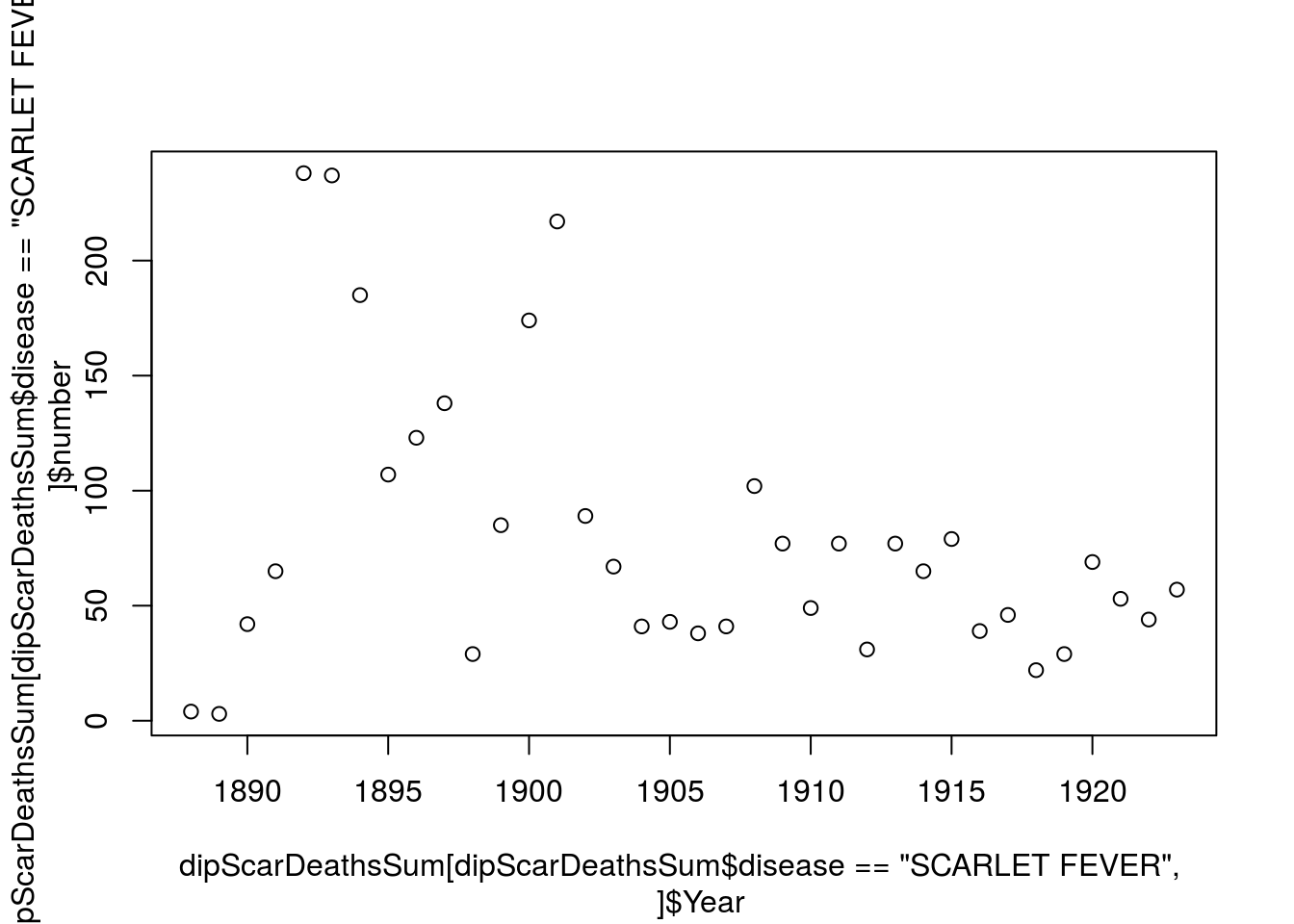The default plot by calling `plot()` is a scatter plot which might not be the best way to represent this data so we can use `type=` to change the type of plot, some options are “l” for line plot, “b” for both a line and scatter plot, and “h” for bars similar to a histogram, lets go with the last one

``````plot(dipScarDeathsSum[dipScarDeathsSum\$disease == "SCARLET FEVER",]\$Year,
dipScarDeathsSum[dipScarDeathsSum\$disease == "SCARLET FEVER",]\$number,
type = "h")  ``````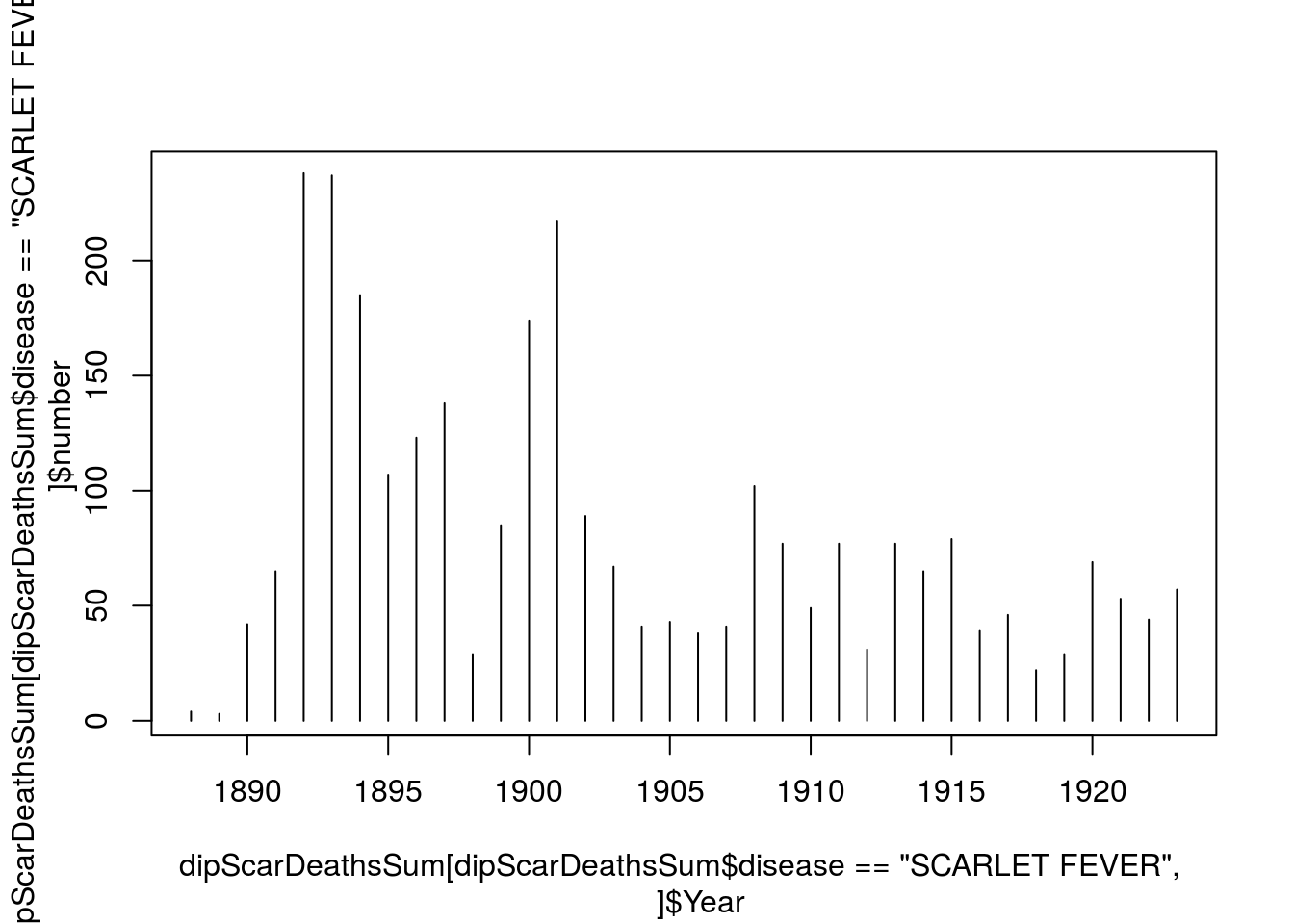Now the default labels look all messed up so lets change them using `xlab=` and `ylab=` and we can add a title as well by using `main=` (which stands for main title)

``````plot(dipScarDeathsSum[dipScarDeathsSum\$disease == "SCARLET FEVER",]\$Year,
dipScarDeathsSum[dipScarDeathsSum\$disease == "SCARLET FEVER",]\$number,
type = "h", xlab = "Year", ylab = "Number of Deaths",
main = "Deaths by SCARLET FEVER")  ``````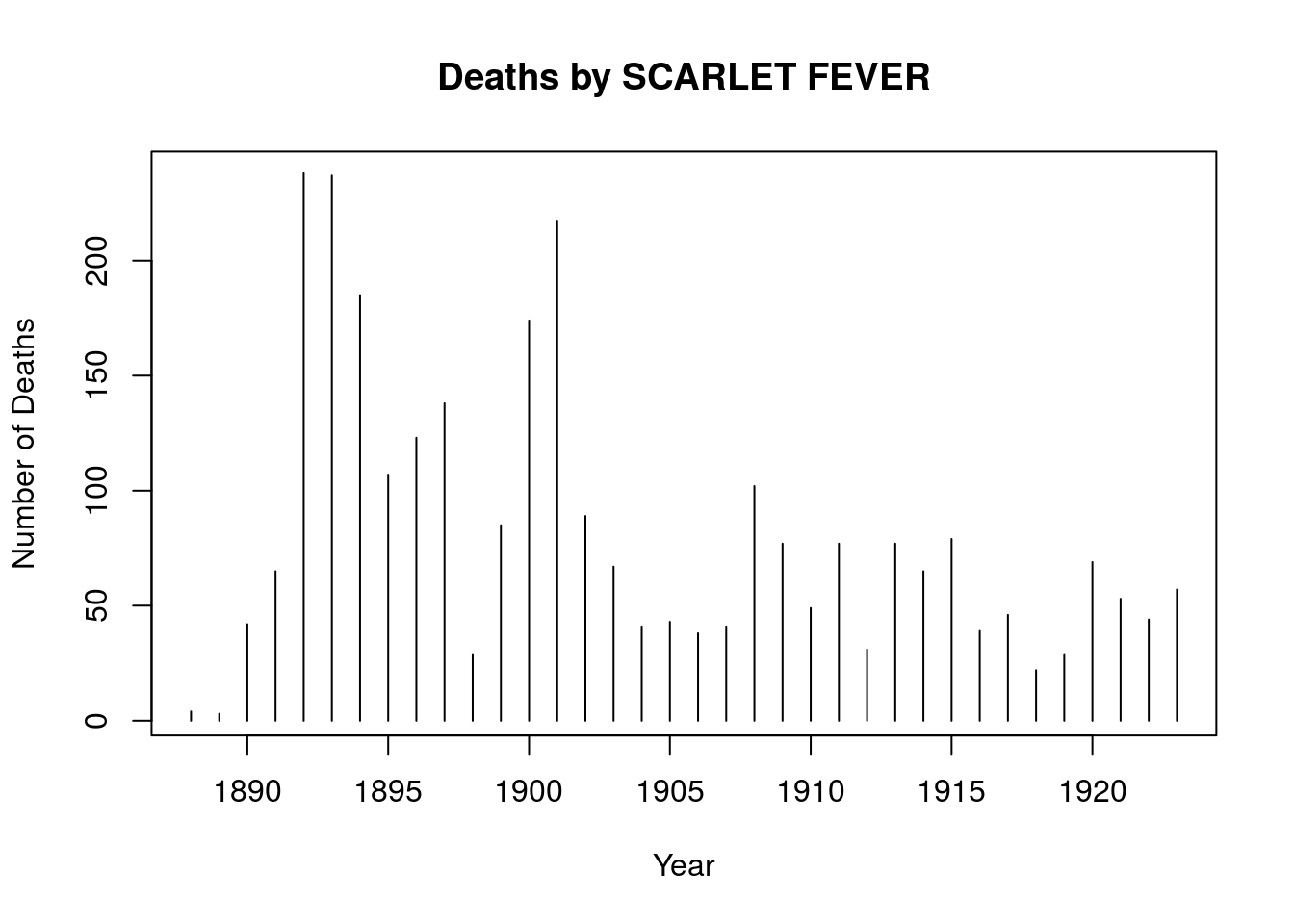Now lets takes away the frame because it doesn’t add much using `bty=` (which stands for box type) and make the lines thicker as well by using `lwd=` (which stands for line width)

``````plot(dipScarDeathsSum[dipScarDeathsSum\$disease == "SCARLET FEVER",]\$Year,
dipScarDeathsSum[dipScarDeathsSum\$disease == "SCARLET FEVER",]\$number,
type = "h", xlab = "Year", ylab = "Number of Deaths",
main = "Deaths by SCARLET FEVER", bty = "none", lwd = 2)  ``````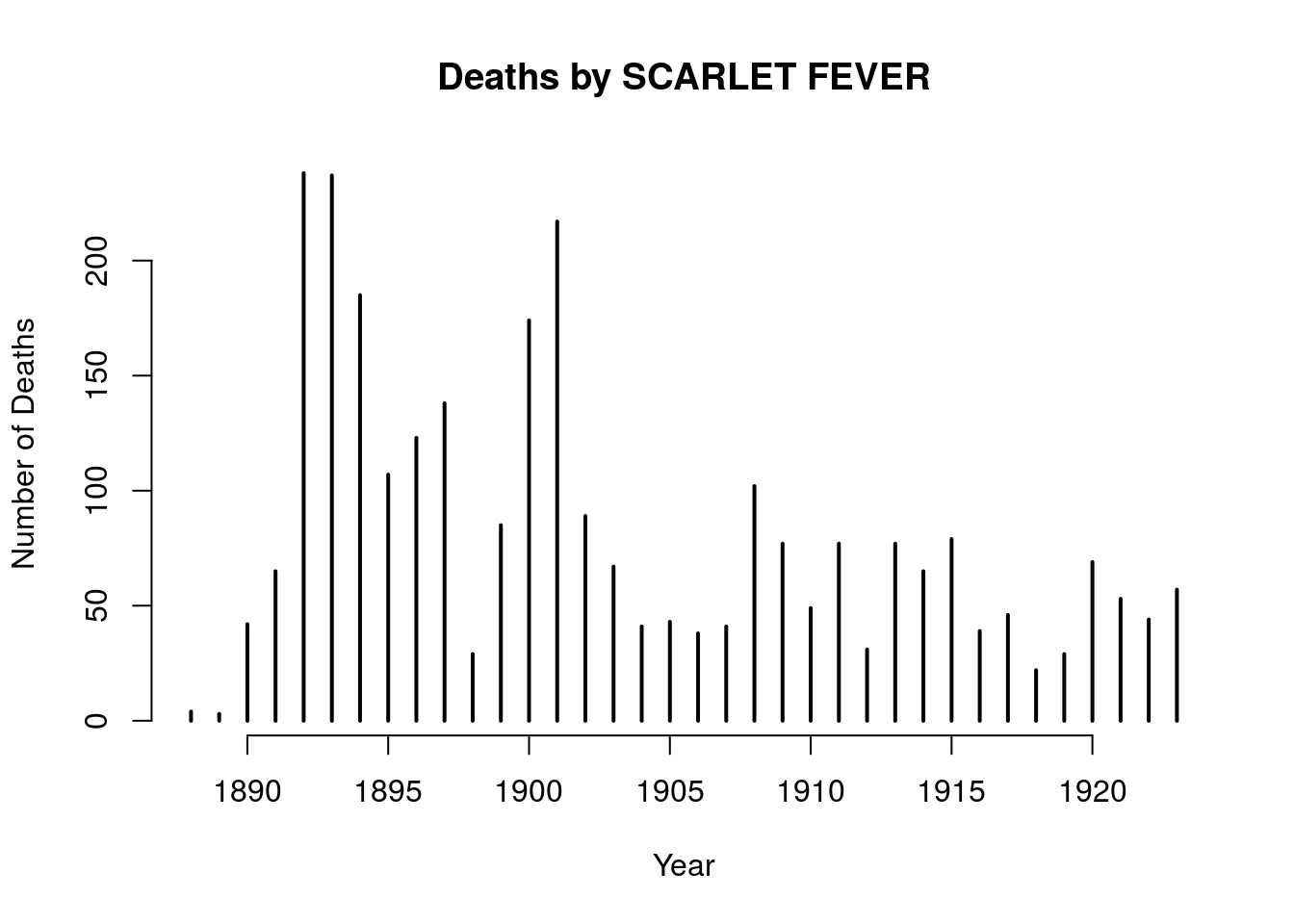Lets add on the data points from the deaths of scarlet fever by using the `points()` function, which is just like the `plot()` function but puts it’s points over the current plot rather than creating a new plot and since we are just adding the points we just need to tell it the type and the line width. Also since we want to see the two data points next to each other, let’s offset the year by 0.5 so the points end up next to each other. Also let’s add color by using the `col=` function

``````plot(dipScarDeathsSum[dipScarDeathsSum\$disease == "SCARLET FEVER",]\$Year,
dipScarDeathsSum[dipScarDeathsSum\$disease == "SCARLET FEVER",]\$number,
type = "h", xlab = "Year", ylab = "Number of Deaths",
main = "Deaths by Diphtheria vs Deaths by Scarlet Fever",
bty = "none", lwd = 2, col = "red")

points(dipScarDeathsSum[dipScarDeathsSum\$disease == "DIPHTHERIA",]\$Year + 0.5,
dipScarDeathsSum[dipScarDeathsSum\$disease == "DIPHTHERIA",]\$number,
type = "h", lwd = 2, col = "darkblue") ``````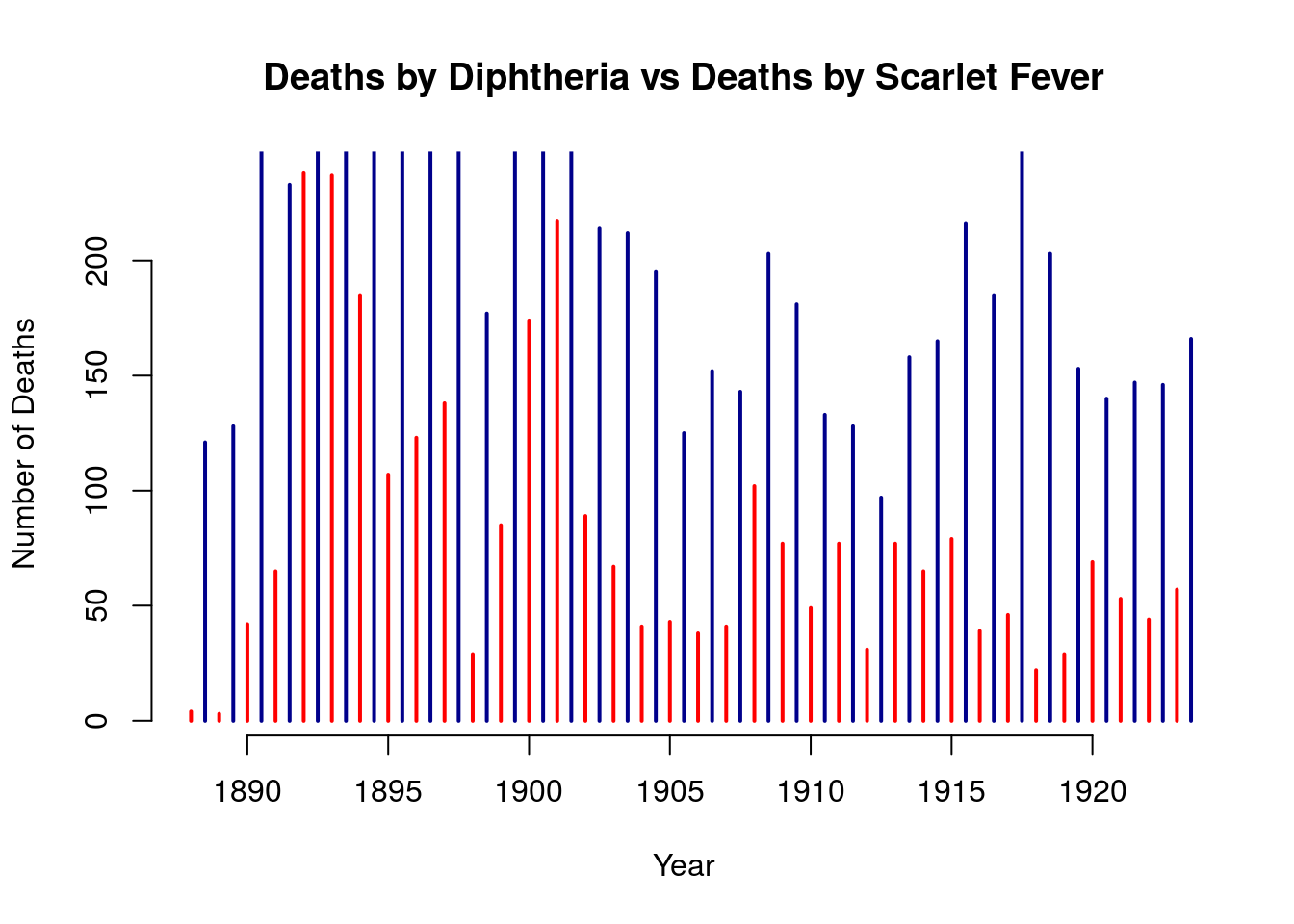It looks like the data points for deaths by Diphtheria are being cut off because the default limites were set by the max number of Scarlet Fever deaths so lets change the xlimits and ylimits by `xlim=c()` and `ylim=c()` to tell the plot to span certain limits. We can also take advantage of the R function `range()` For example if we call `range()` on the numbers column we can get the max number and min numbers

``range(dipScarDeathsSum\$number)``
``   3 837``
``````plot(dipScarDeathsSum[dipScarDeathsSum\$disease == "SCARLET FEVER",]\$Year,
dipScarDeathsSum[dipScarDeathsSum\$disease == "SCARLET FEVER",]\$number,
type = "h", xlab = "Year", ylab = "Number of Deaths",
main = "Deaths by Diphtheria vs Deaths by Scarlet Fever",
bty = "none", lwd = 2, xlim = range(dipScarDeathsSum\$Year),
ylim = range(dipScarDeathsSum\$number), col= "red")

points(dipScarDeathsSum[dipScarDeathsSum\$disease == "DIPHTHERIA",]\$Year + 0.5,
dipScarDeathsSum[dipScarDeathsSum\$disease == "DIPHTHERIA",]\$number,
type = "h", lwd = 2, col = "darkblue") ``````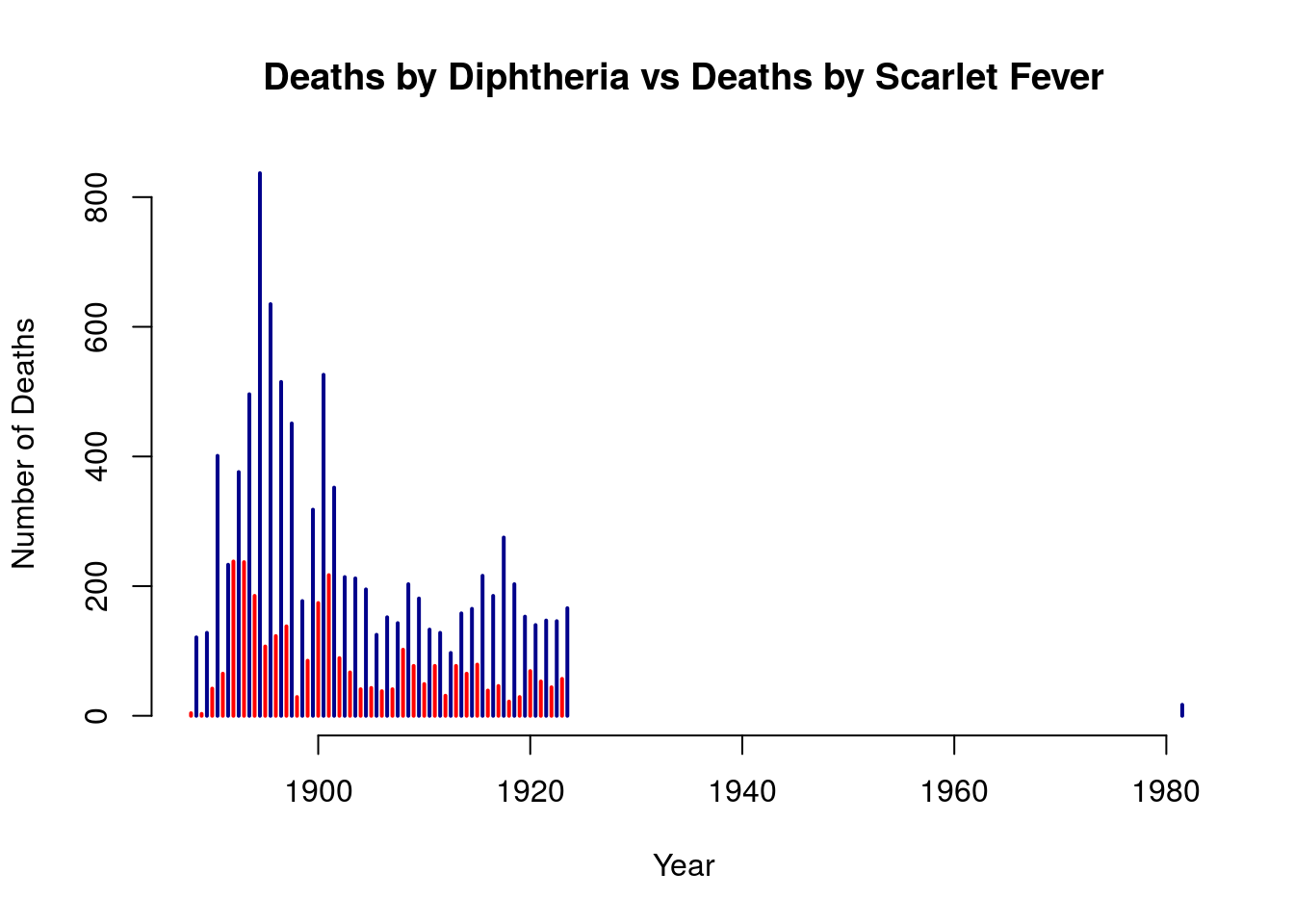Since there isn’t much data after the 1920 it might be more interesting to look at only the data through that time point so we can see the data in more detail, lets change the `xlim=c()` to the change the year range

``````plot(dipScarDeathsSum[dipScarDeathsSum\$disease == "SCARLET FEVER",]\$Year,
dipScarDeathsSum[dipScarDeathsSum\$disease == "SCARLET FEVER",]\$number
, type = "h", xlab = "Year", ylab = "Number of Deaths",
main = "Deaths by Diphtheria vs Deaths by Scarlet Fever",
bty = "none", lwd = 2, xlim =c(1880, 1930),
ylim = range(dipScarDeathsSum\$number), col= "red")

points(dipScarDeathsSum[dipScarDeathsSum\$disease == "DIPHTHERIA",]\$Year + 0.5,
dipScarDeathsSum[dipScarDeathsSum\$disease == "DIPHTHERIA",]\$number,
type = "h", lwd = 2, col = "darkblue") ``````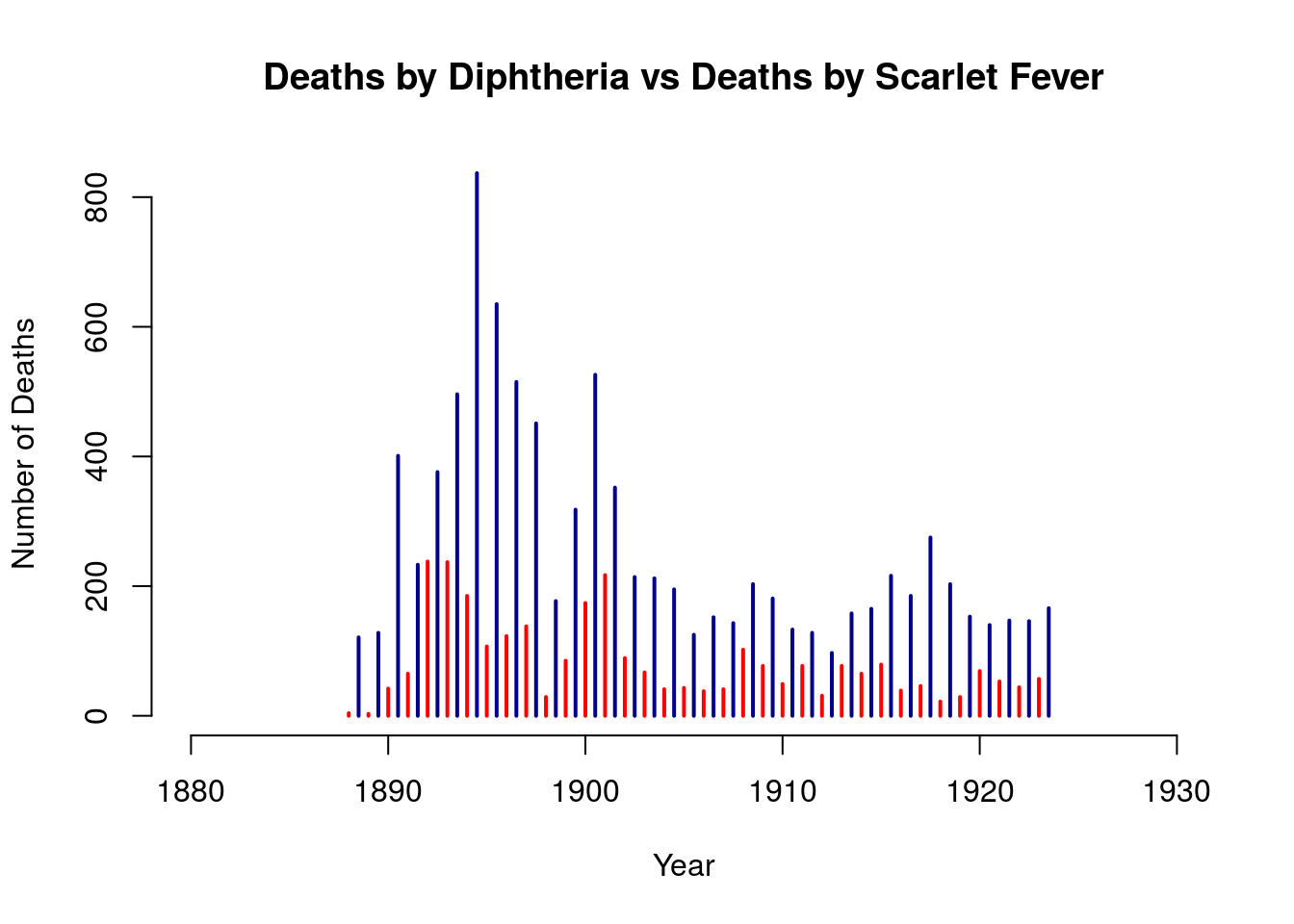We can also add a legend

``````plot(dipScarDeathsSum[dipScarDeathsSum\$disease == "SCARLET FEVER",]\$Year,
dipScarDeathsSum[dipScarDeathsSum\$disease == "SCARLET FEVER",]\$number,
type = "h", xlab = "Year", ylab = "Number of Deaths",
main = "Deaths by Diphtheria vs Deaths by Scarlet Fever",
bty = "none", lwd = 2, xlim =c(1880, 1930),
ylim = range(dipScarDeathsSum\$number), col= "red")

points(dipScarDeathsSum[dipScarDeathsSum\$disease == "DIPHTHERIA",]\$Year + 0.5,
dipScarDeathsSum[dipScarDeathsSum\$disease == "DIPHTHERIA",]\$number,
type = "h", lwd = 2, col = "darkblue")

legend("topright", legend = c("SCARLET FEVER", "DIPHTHERIA"),
lwd = 2, col = c("red", "darkblue"))``````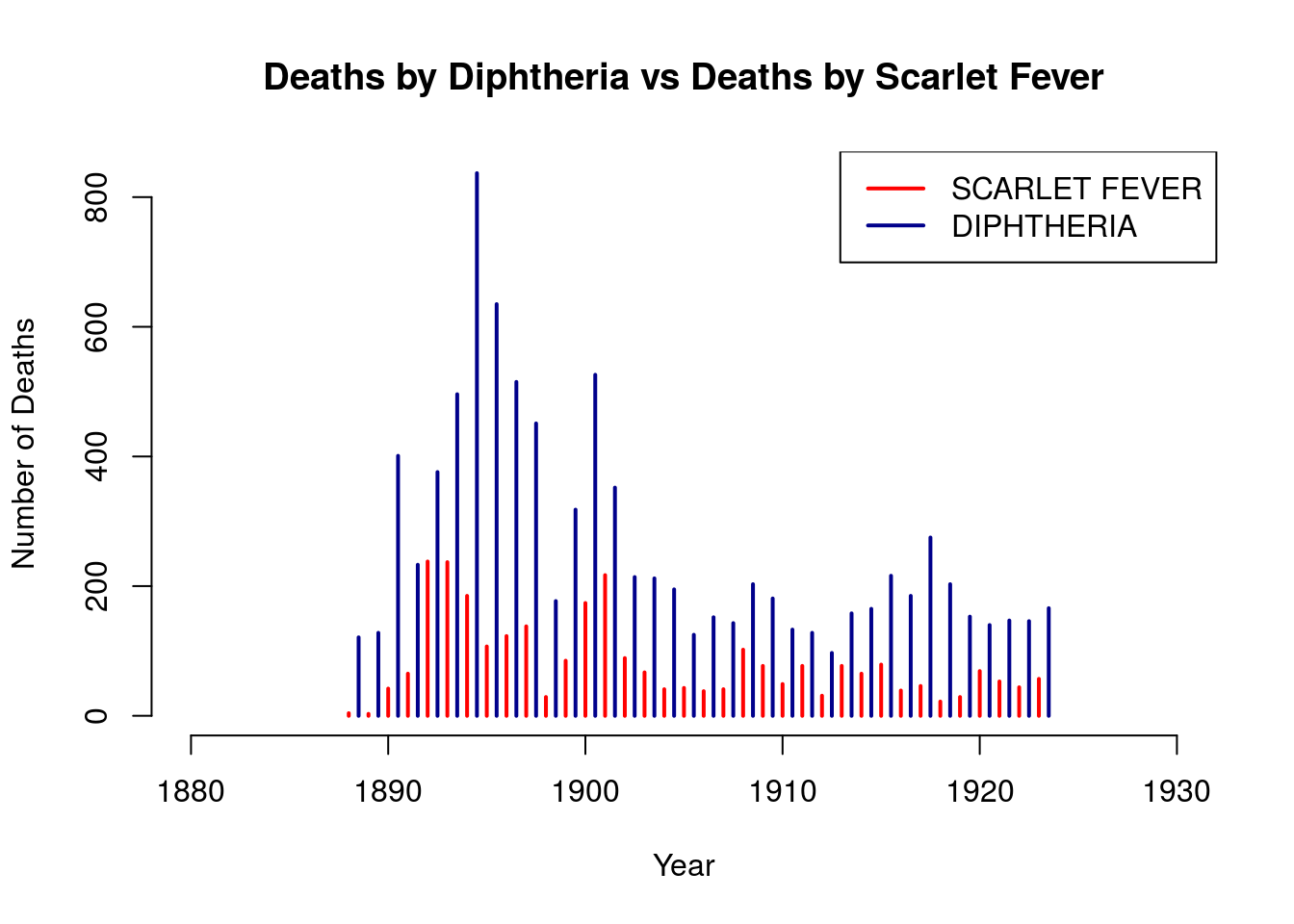## Part 3 Exercises

1. Try customizing some plots for the diseases found in the Worcester Epi Dataset
2. For example trying to look how deaths have decreased over time for some diseases like measles (though maybe on the raise again), smallpox, etc
3. Try creating a plot comparing deaths between SCARLET FEVER and TYPHOID FEVER [ENTERIC FEVER]
4. Try ploting a couple of the big diseases in the 1920’s

Now we can use the colors provided by R but we can also use an R package called `RColorBrewer` or `colorspace` to provide us with even more colors but first we have to learn how to download the packages which can be done using `install.packages()` and `library()` or we can use RStudio to download them

Using `install.packages()` and `library()`

``````#install package
install.packages("RColorBrewer")
#Once package is installed to actually use it you need to load it
library("RColorBrewer")``````

Or we can use RStudio to manually install the packages
In the bottom right corner of RStudio click the Packages tab and then the install icon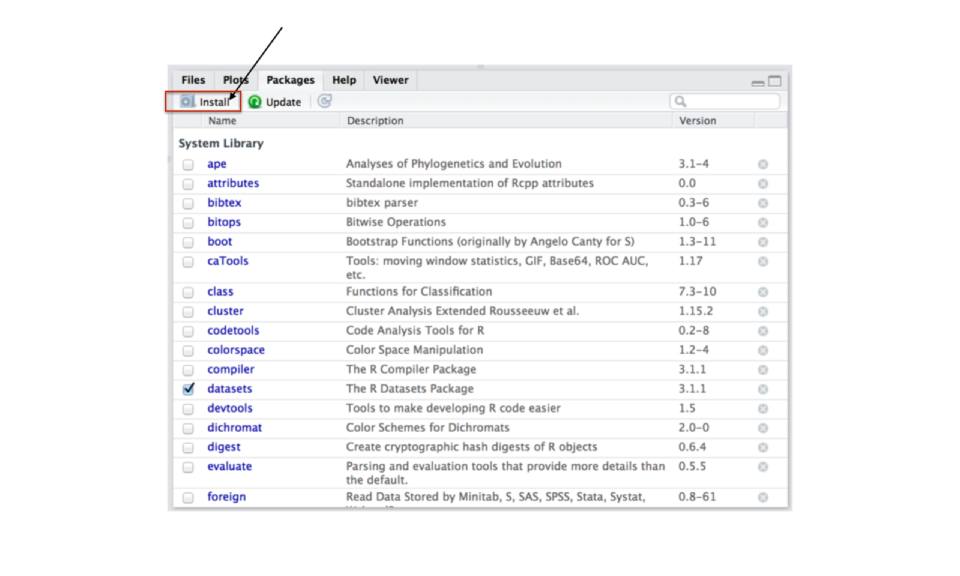Type in RColorBrewer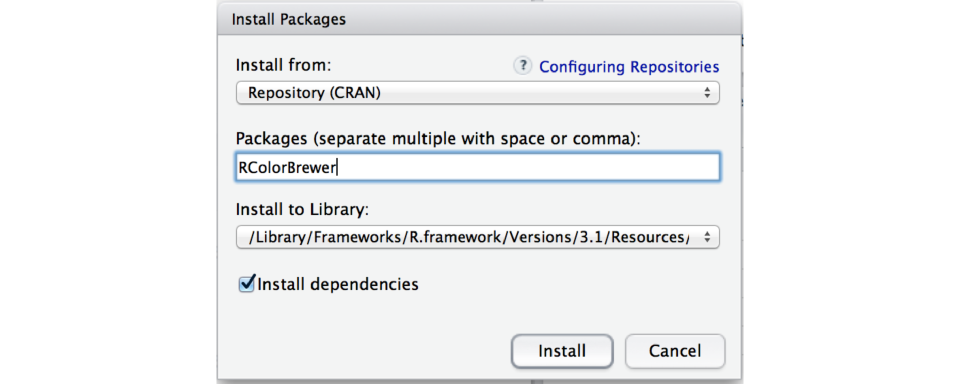To Load click the check box next to RColorBrewer